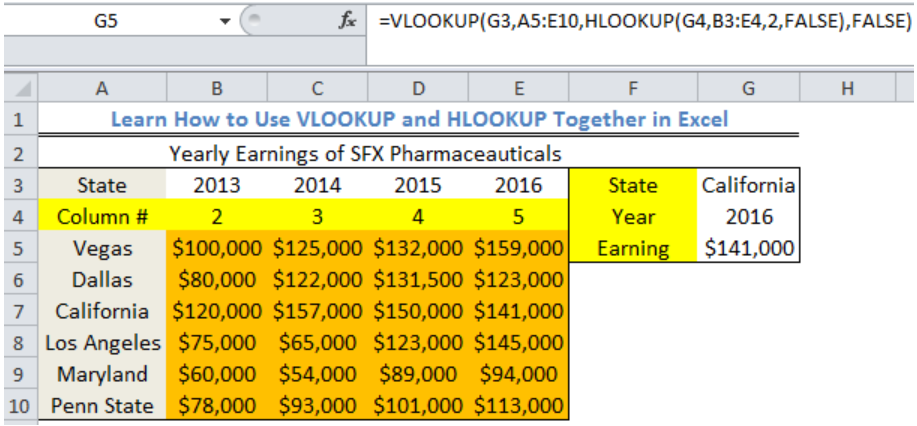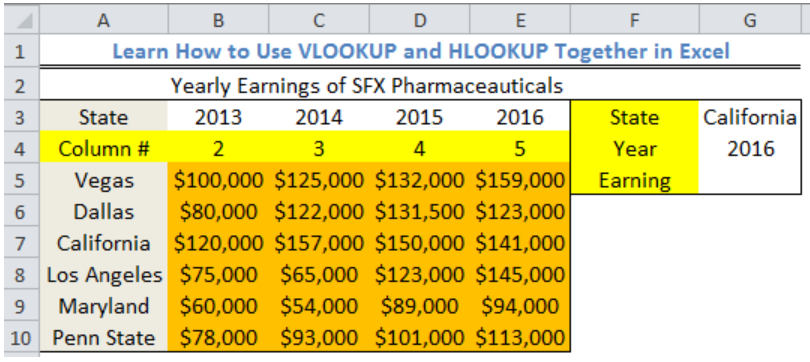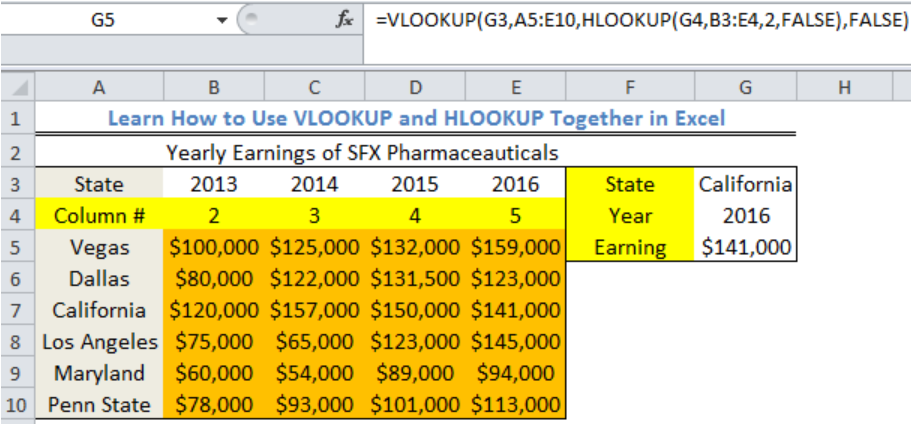Get instant live expert help with Excel or Google Sheets“My Excelchat expert helped me in less than 20 minutes, saving me what would have been 5 hours of work!”

#### Post your problem and you’ll get expert help in seconds.

Your message must be at least 40 characters
Our professional experts are available now. Your privacy is guaranteed.

# Learn How to Use VLOOKUP and HLOOKUP Together in Excel

We can use a nested formula that combines the VLOOKUP and HLOOKUP Functions in excel to retrieve values from a table. Approximate and exact matching is supported by this combined formula and wildcards (* ?) are for finding partial matches. The steps below will walk through the process.Figure 1- How to Use VLOOKUP and HLOOKUP Together in Excel

## Syntax

`=VLOOKUP(lookup_value,lookup_array,HLOOKUP(lookup_value ,lookup_array,2,range_lookup),range_lookup)`

• Lookup_Value: This is the value to search for
• Lookup_array: This is the range to search for the lookup value
• HLOOKUP: This serves as the COLUMN NUMBER in the VLOOKUP formula. It specifies the COLUMN where we retrieve the data
• Range_lookup: This is used to specify if we want an approximate or exact match. If omitted, an approximate match is used

## Formula

`=VLOOKUP(G3,A5:E10,HLOOKUP(G4,B3:E4,2,FALSE),FALSE)`

## Setting up the Data

• We will use the combined formula to find the earning of California in 2016.Figure 2 – Setting up the Data

## Using the VLOOKUP and HLOOKUP Functions

• We will click on Cell G5
• We will insert the formula below into Cell G5
`=VLOOKUP(G3,A5:E10,HLOOKUP(G4,B3:E4,2,FALSE),FALSE)`
• We will press the enter keyFigure 3- Result of the VLOOKUP and HLOOKUP Functions

## Explanation

In this formula, the horizontal value (2016) is looked up with the HLOOKUP section of the formula. The returned value by HLOOKUP is 5 because HLOOKUP searches for 2016 in the range B3:E4 in ROW 2.

The vertical lookup value (California) is searched for by VLOOKUP. VLOOKUP searches the range A5:E10 for what is contained in Cell G3. After identifying California in the range, it then matches California with the returned column value (5) by HLOOKUP and returns \$141,000.

## Note

• If the lookup_value is greater than every value in the lookup table, the formula returns with the last value provided it has been set to approximate match
• If the lookup_value is less than all values in the lookup table, the function returns the #N/A error
• If we omit range_lookup, both functions will allow a non-exact or approximate match, but it will use an exact match if one exists. The approximate match is set as 1 and exact match is set as 2.
• If we set the range_lookup to approximate match, then the lookup values in the first row of the table must be sorted in ascending order. Otherwise, HLOOKUP may return an incorrect or unexpected value.
• If range_lookup is FALSE (exact match), values in the first row of the table do not need to be sorted.

This formula can only be used when the data is in a matrix form.

## Instant Connection to an Expert through our Excelchat Service

Most of the time, the problem you will need to solve will be more complex than a simple application of a formula or function. If you want to save hours of research and frustration, try our live Excelchat service! Our Excel Experts are available 24/7 to answer any Excel question you may have. We guarantee a connection within 30 seconds and a customized solution within 20 minutes.

Solution examplesI'm looking for a formula or format for if there is data in c4 on a sheet... It adds extra information underneath
Solved by X. E. in 20 minsI have an excel spreadsheet with two worksheets. And the following formula is not pulling the data. =VLOOKUP(\$A\$2:\$A\$566,'Module Type Info'!\$A\$2:\$D\$97,4,FALSE) In sheet 1, I am using all data in column 1 for the lookup In sheet 2, I have selected the first 4 columns of data for array I want to pull data from sheet 2, column 4 into sheet 1 Both tabs are sorted alphabetically. Confirmed that the value in column 4 of sheet 2 is a TEXT field. Why is this not pulling the info from sheet 2 into sheet 1
Solved by F. L. in 39 minsI am trying to perform a vlookup or index match for a large data set. Normally I rely on vlookup but it is giving me data from the wrong column whatever I do. I have tried to move to vlookup and it is doing exactly the same thing. Do you have any tips?
Solved by O. W. in 27 minsHi, I have a problem with vlookup formula, I am not sure what am I doing wrong but it seems to not be working
Solved by K. F. in 40 minsI need help with Vlookup- am trying to copy a data from one sheet onto another matching the number but with a column
Solved by C. H. in 20 mins